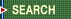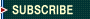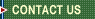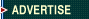September 25, 2023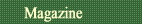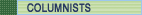Quality Applications SPC Guide First Word Letters What Works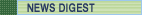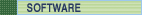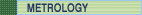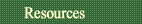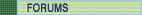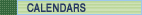Davis Balestracci

## Why Three Standard Deviations?

Analysis of means offers a way to address real improvements.

My last three columns have demonstrated a statistical approach to stratification known as analysis of means (ANOM), invented by the late Ellis Ott and explained beautifully in his book, Process Quality Control (Fourth Edition, ASQ Quality Press, 2005). My experience is that ANOM is woefully underutilized, and I think it should be a bread-and-butter tool of any statistical practitioner.

Most required statistics courses give the impression that two standard deviations ("95-percent confidence") is the gold standard of comparison. However, you might notice that in most academic exercises, only one decision is made. The analyses in my columns made multiple, simultaneous decisions: six in the November 2005 column ("Taking Count Data a Step Further, Part 1"), five in the December 2005 column ("Taking Count Data a Step Further, Part 2") and 51 in last month's column ("Are You Using SWAGs?").

All three of these situations represent systems where, theoretically, there should be no differences among the units being compared. It's not as if these represented experiments where each unit was assigned a different treatment, and one was intentionally looking for significant differences. The assumption is that all units being compared are equivalent until proven other-wise, and they represent a system with an average performance. Each unit's performance will also be considered average unless the data show otherwise.

Given that, suppose one used the common, two-standard-deviation comparison? One must now consider, if there were no differences among the units, what's the probability that all of them would be within two standard deviations? The answer is (0.95) n, where "n" is the number of units being simultaneously compared. For our three examples, (0.95) 6 = 0.735, (0.95) 5 = 0.774 and (0.95) 51 = 0.073.

In other words, using two standard deviations means that one would run the following risks of accidentally declaring at least one of the units as an outlier when it actually isn't: 100% - 73.5% = 26.5 percent and, similarly, 22.6 percent and 92.7 percent, respectively.

To more precisely determine a 5-percent risk, one must use the Z- (i.e., normal distribution) or t-value corresponding to an overall probability risk of 0.05, which turns out to be: e [ln(0.975)/n]. For our three scenarios, one would need to use limits comparable not to 0.95, but 0.996, 0.995 and 0.9995, respectively. (Note: 0.996 6 ~ 0.975, 0.995 5 ~ 0.975, 0.9995 51 ~ 0.975.)

Some of you are wondering, "Why are you using 0.975 in the calculation and not 0.95?" Because I'm using a two-sided test (which, by the way, is how you obtained the "two" in two standard deviations in the first place). Just let me be the statistician for a moment because this will all turn out to be moot!

Let's just keep things simple and use the normal approximation. This corresponds to Z-scores of 2.63, 2.57 and 3.29, respectively.

If this wasn't bad enough, it now gets even more complicated. Ideally, in considering each unit as a potential outlier, wouldn't you like to eliminate each data point's influence on the overall calculations, replot the data and see whether they now fall within the remaining units' system? Actually, there's a statistical sleight of hand that does this by adjusting these limits via a factor utilizing the "weight" of each observation. For the simplest case of equal sample sizes, this becomes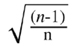,

where "n" is the number of units being compared.

If our units had equal sample sizes (and they don't), the actual limits used for an overall risk of 5 percent would be 2.41, 2.30 and 3.26, respectively.

If I were to do a statistically exact analysis for every unit, I would actually have to calculate different limits for each because the sample sizes aren't equal. In this case, the adjustment factor becomes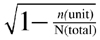.

Here's my point: For those of you familiar with Deming's famous red bead experiment, remember that in comparing the performance of the "willing workers" he actually uses three-standard-deviation limits. He also makes a comment in Out of the Crisis (MIT CAES, 1982) that using exact probability limits such as I'm calculating isn't proper, although he really doesn't explain why.

Brian Joiner, a former student of Ott, told me that Ott himself used three-standard-deviation limits. In other words, when looking for opportunities for improvement, i.e., exposing inappropriate or unintended variation, using three standard deviations along with an estimate of the standard deviation that's calculated appropriately is "good enough."

If you wanted to approximate the overall risk you're taking in using three standard deviations, I suppose you could calculate 1 - (0.997) n (the 0.997 famous from control-chart theory, which uses three standard deviations), which translates to risks of 1.8 percent, 1.5 percent and 14.4 percent, respectively, for our n = 6, 5 and 51. This is conservative in the first two cases and more risky when comparing the 51 physicians, but it gets the conversation started.